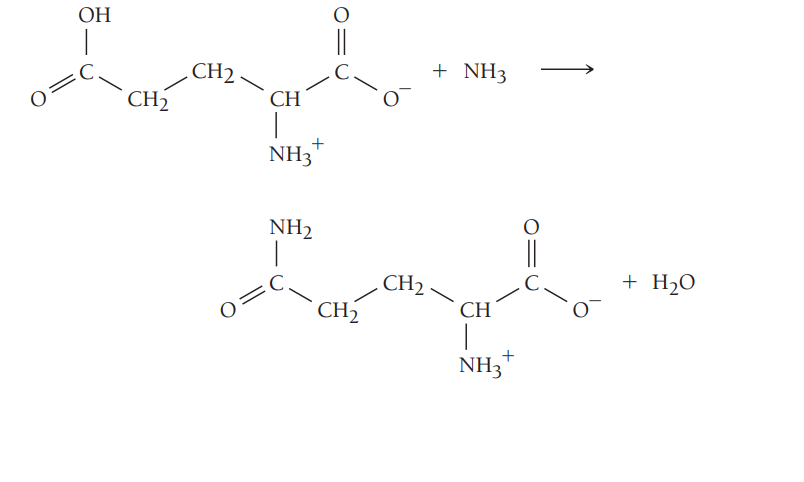×
Get Full Access to Chemistry: A Molecular Approach - 5 Edition - Chapter 19 - Problem 103
Get Full Access to Chemistry: A Molecular Approach - 5 Edition - Chapter 19 - Problem 103

×ISBN: 9780134874371 2047

## Solution for problem 103 Chapter 19

Chemistry: A Molecular Approach | 5th Edition

• Textbook Solutions
• 2901 Step-by-step solutions solved by professors and subject experts
• Get 24/7 help from StudySoup virtual teaching assistantsChemistry: A Molecular Approach | 5th Edition

4 5 1 240 Reviews
25
0
Problem 103

Review the box in this chapter entitled Chemistry in Your Day: Making a Nonspontaneous Process Spontaneous. The hydrolysis of ATP, shown in Problem 91, is often used to drive nonspontaneous processes-such as muscle contraction and protein synthesis-in living organisms. The nonspontaneous process to be driven must be coupled to the ATP hydrolysis reaction. For example, suppose the nonspontaneous process is $$\mathrm{A}+\mathrm{B} \longrightarrow \mathrm{AB}$$ ( $$\Delta G^{\circ}$$ positive). The coupling of a nonspontaneous reaction such as this one to the hydrolysis of ATP is often accomplished by the mechanism:

$$\mathrm{A}+\mathrm{ATP}+\mathrm{H}_{2} \mathrm{O} \longrightarrow \mathrm{A}-\mathrm{P}_{\mathrm{i}}+\mathrm{ADP}$$

$$\mathrm{A}-\mathrm{P}_{\mathrm{i}}+\mathrm{B} \longrightarrow \mathrm{AB}+\mathrm{P}_{\mathrm{i}}$$

$$\overline{\mathrm{A}+\mathrm{B}+\mathrm{ATP}+\mathrm{H}_{2} \mathrm{O} \longrightarrow \mathrm{AB}+\mathrm{ADP}+\mathrm{P}_{\mathrm{i}}}$$

As long as $$\Delta G_{\mathrm{rxn}}^{\circ}$$ for the nonspontaneous reaction is less than 30.5 kJ, the reaction can be made spontaneous by coupling in this way to the hydrolysis of ATP. Suppose that ATP is to drive the reaction between glutamate and ammonia to form glutamine:a. Calculate K for the reaction between glutamate and ammonia. (The standard free energy change for the reaction is +14.2 kJ/mol. Assume a temperature of 298 K.)

b. Write a set of reactions such as those given showing how the glutamate and ammonia reaction can couple with the hydrolysis of ATP. What are $$\Delta G_{\text {rxn }}^{\circ}$$ and K for the coupled reaction?

Text Transcription:

A + B longrightarrow AB

Delta G^circ

A + ATP + H2O longrightarrow A - Pi + ADP

A-Pi + B longrightarrow AB + Pi

A + B + ATP + H2O longrightarrow AB + ADP + Pi

Delta G_rxn^circ

Step-by-Step Solution:
Step 1 of 3

9/19 ­ 4.1 and 4.2: Alkene/Alkyne Naming, Cis/Trans, E/Z ● Unsaturated hydrocarbons ○ C­C double bond, triple bond, or benzene­like ring (alkene) (alkyne) ● Structure ○ Alkene = 120​ bond o​ ○ Alkyne = 180​ bond ○ Can’t rotate about a triple or double bond ● Nomenclature ○ Parent chain must include double/triple bond ○ Double/triple bond gets lowest # ○ double/triple bond locant goes before ­ene/­yne ○ ­ene listed before ­yne ● Isomers ○ Cis ­ groups on same side of double bond or ring Trans ­groups on opposite side of double bond or ring ■ Cis is only stable isomers for cyclic alkene w/ less than 8 atoms ○ Disubstituted cyclohexane ○ EZ notation ■ Z ­ groups of high priority on same side of bond or ring ­­ (on ze zame zide) E ­ groups of high priority on opposite side of bond or ring ■ Priority ● Higher atomic # = higher priority 9/22 ­ 5.1: Energy Diagrams ● Alkene Reactions ○ Compounds react across double bond ○ Rxns require energy input ● Enthalpy ­ △H, q ­ heat energy exchange ○ Breaking bonds requires energy absorption ○ Most rxns involve multiple bonds ○ Endothermic = energy enters system ○ Exothermic = energy leaves system

Step 2 of 3

Step 3 of 3

## Discover and learn what students are asking

Statistics: Informed Decisions Using Data : Discrete Random Variables
?What are the two requirements for a discrete probability distribution?

Unlock Textbook Solution